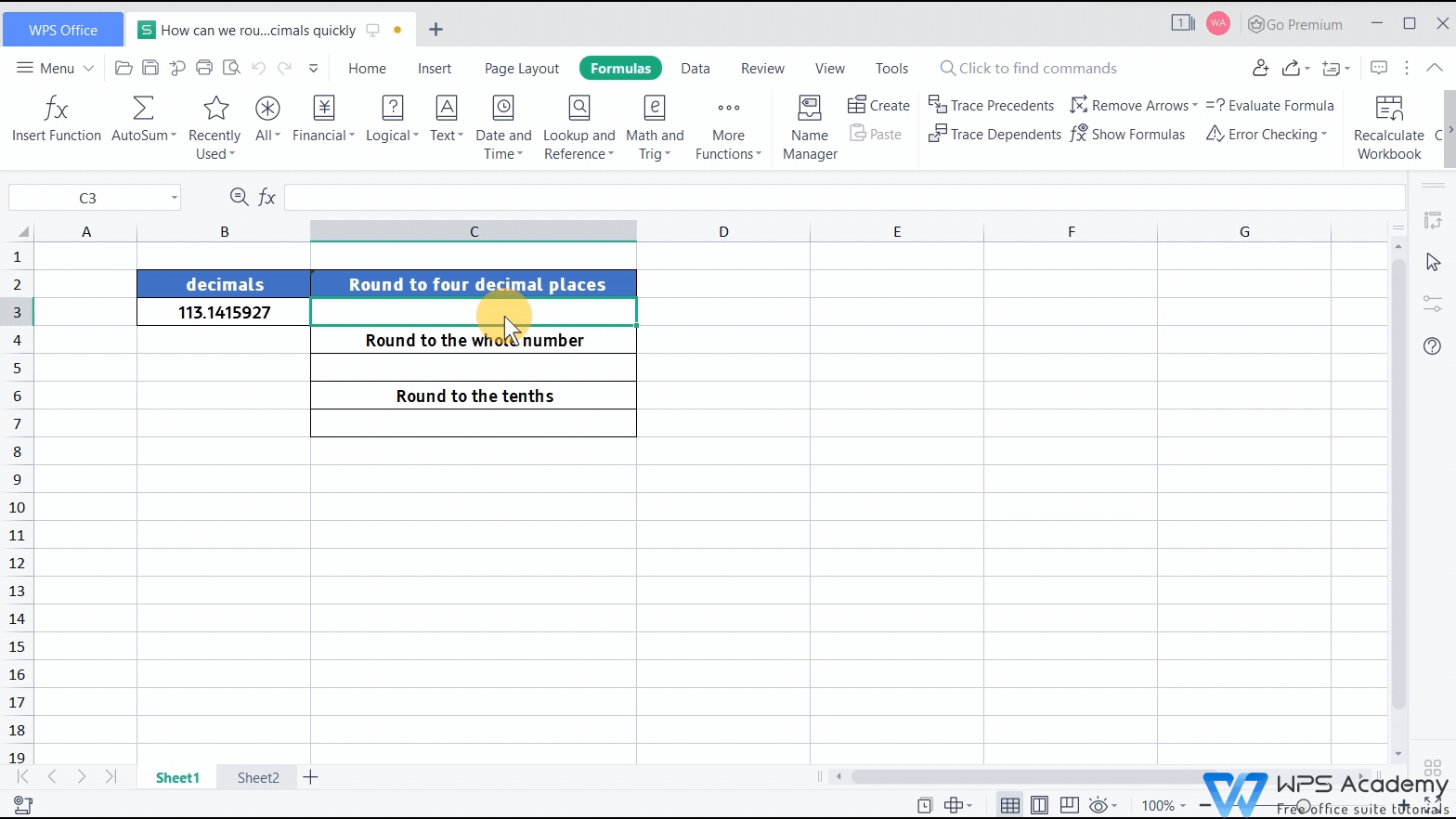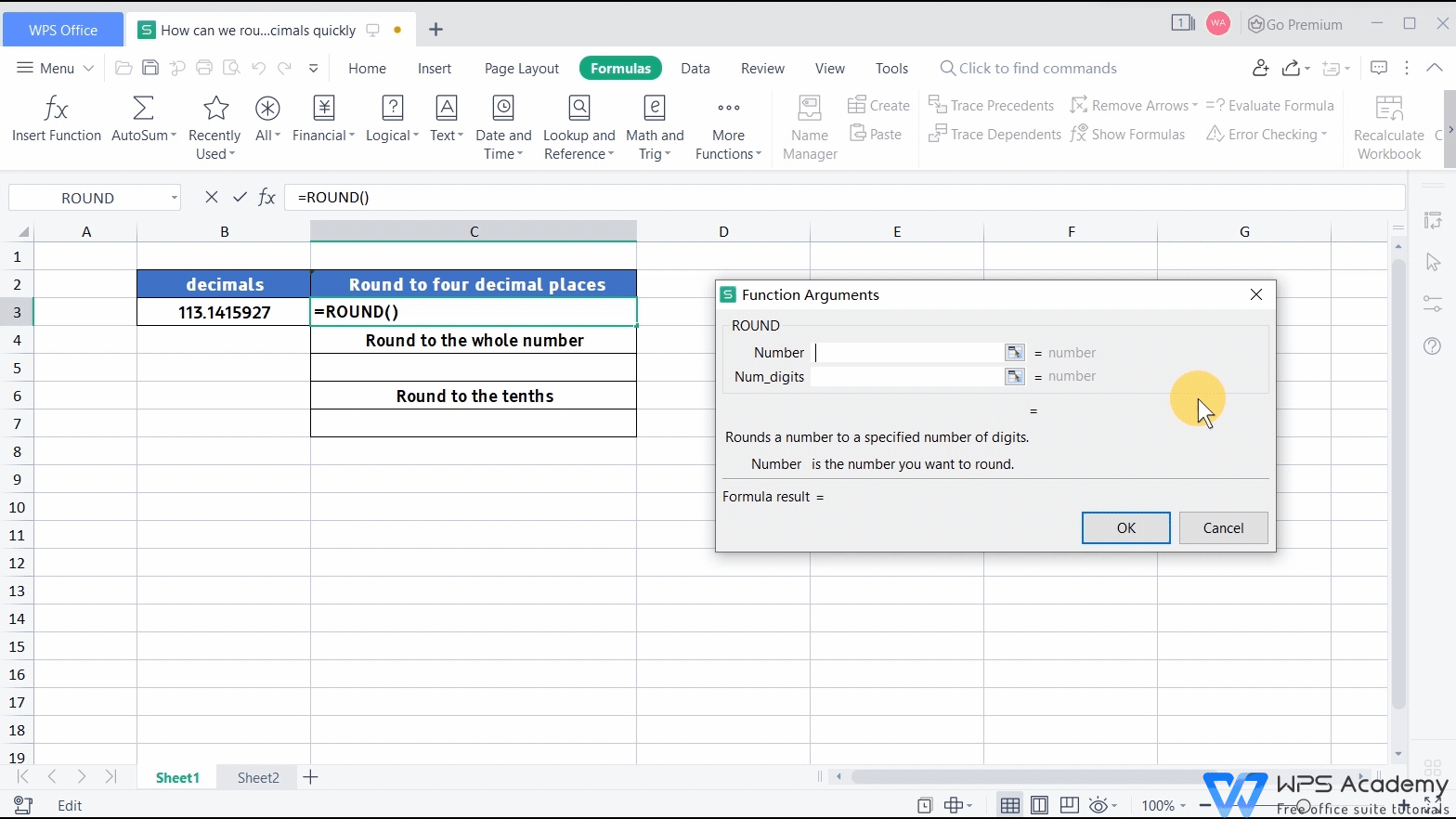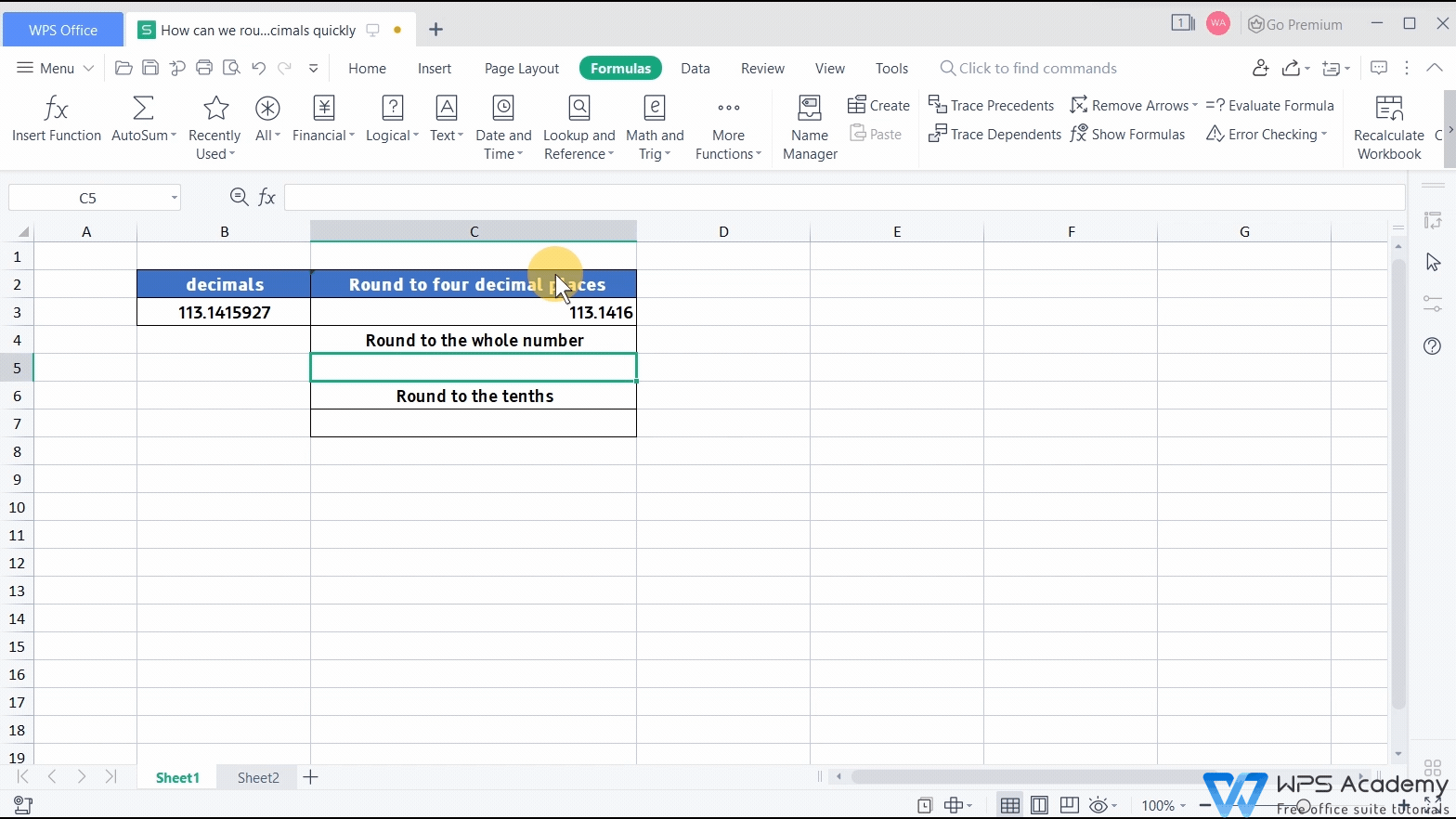# Use the ROUND function to round decimals quickly### Graphic skills

In our work and study, we often have to round off decimals. Let me show you how to use the ROUND function to deal with long strings of decimals quickly.

Take the decimal in the B3 cell as an example.

1. Place the cursor in C3.

2. Click Insert Function.

3. In Math and Trig, select the ROUND function.The pop-up dialog box has two parameters. Number is the numerical value to be rounded, and Num_digits refers to the digits to be used for rounding.

If the Num_digits is greater than 0, then the number will be rounded to the specified number of Num_digits. If the Num_digits equals 0, then the number will be rounded to the nearest integer. If the Num_digits is less than 0, the number will be rounded to the corresponding digit to the left of the decimal point.

In the Number in the selection of the B3 cell, because we want to round to four decimal places, we enter 4 in the Num_digits, and click OK.Select the C5 cell, insert the ROUND function. Because we want to round to the whole number, enter 0 in Num_digits and click OK finally.Select the C7 cell, insert the ROUND function. Because we want to round to the second place to the left of the decimal point, we enter -2, and then click OK.So in practical work, how can we use the ROUND function?

Take this payroll as an example. We need to round the after-tax income to two decimal places.

1. We need to select the G3 cell and insert the ROUND function.

2. Enter F3 and 2 for Number and Num_digits, respectively.

3. Click OK.What a fast and quick function! Easy your work with WPS Spreadsheet like Microsoft Excel. Did you get it?